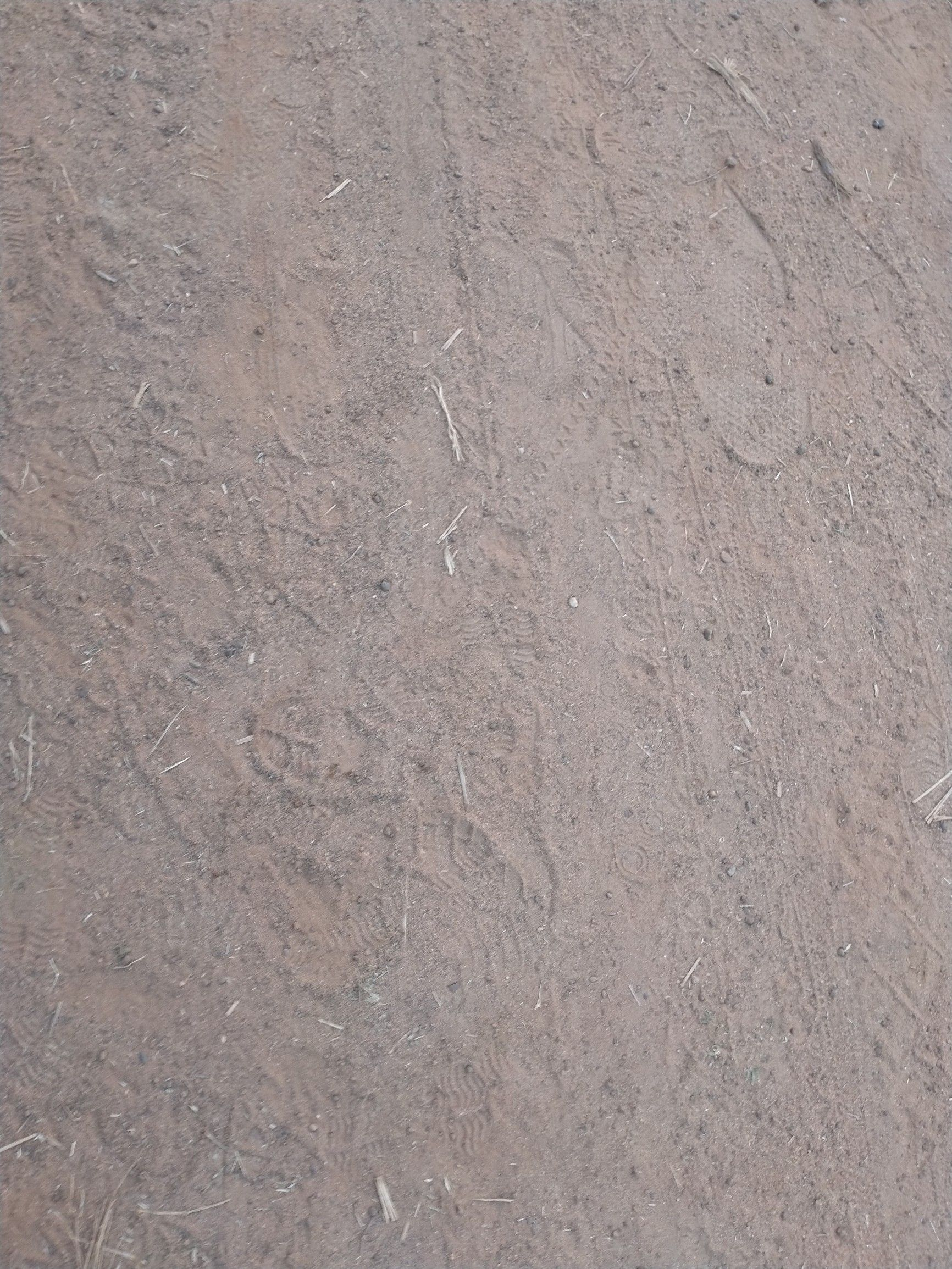Symbol
ProblemLearning Task 2 A. Determine the factors of the given polynomial. - $1.x3+2x2-5x-6$ $2.x3+x2-x-1$ $3.x3-x2-10x-8$ - - - B. Solve the following problems using factoring polynomials. 1. A rectangular garden in a backyard has an area $of\left(3x2+5x-2\right)$ square me- ters. Its width is (x + 2) meters. a. Find the length of the garden. b. You $dcc$ $dc$ to partition the garden into two or more smaller congruent gardens. $Dcsig1$ a possible model and include mathematical concepts in your design. 2. $1fonc$ $camofbondPapcr$ $or\left(6x4-17x3+24x2$ $cos1s\left(x-4\right)Pcs0s$ $-34x+24\right)pcsos7$ how many reams can you buy for A $carnlng$ Task 3 A. Answer the $011os$ $ing$ 1. What is the remainder when $n2x20+7x3-51s$ divided $byx-12$ 2. What is the remainder $x2+8x$ $+12$ $whcn$ divided $byx-27$ 3. What are the factors $ofx2+8x+127$ 4. If the remainder of the $x3-kox2+3x-2$ when divided by $x-11s$ 4, find the value of k. B. Answering the following questions. 1. How do you factor polynomials? Discuss the mathematics concepts and principles applied when factoring polynomials. 2. What new realizations do you have about factoring polynomials? How would you connect this to real life? How would you use this in making $dccisions7$
10th-13th grade
Other
Search count: 143
SolutionQanda teacher - Ëß\ær